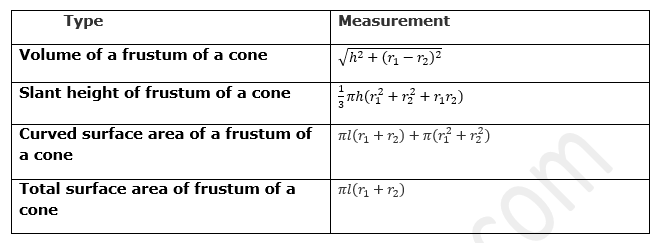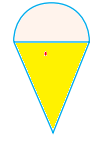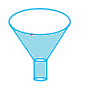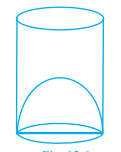# Class 10 Maths Worksheet for Surface Area and Volume

Given below are the Class 10 Maths Worksheet for Surface Area and Volume
a. Multiple choice questions
b. Match the column
c. Calculation problems
d. True and False questions

## Multiple Choice questions

Question 1.
A bucket is in the form of a frustum of a cone ad holds 28.490 liters of water. The radii of the top and bottom are 28cm and 21cm respectively. Find the height of the bucket.
a. 20 cm
b. 15 cm
c. 10 cm
d. None of the above

Question 2.
Lead spheres of diameter 6cm are dropped into a cylindrical beaker containing some water and are fully submerged. If the diameter of the beaker is 18cm and water rises by 40cm. find the no. of lead sphere dropped in the water.
a. 90
b. 150
c. 100
d. 80

Question 3.
A petrol tank is a cylinder of base diameter 21cm and length 18cm fitted with conical ends each of axis length 9cm. Determine the capacity of the tank
a. 8316cm3
b. 9456 cm3
c. 10160 cm3
d. None of the above

## Calculation Problems

Question 4.
A cone, a hemisphere and a cylinder stand on equal bases and have the same height. Show that their volumes are in the ratio 1: 2: 3.

Question 5.
The radii of the basses of two right circular solid cones of same height are r1 and r2 respectively. The cones are melted and recast into a solid sphere of radius R. Show that the height of each cone is given by
$h=\frac {4R^3}{r_1^{2} + r_2^{2}}$

Question 6.
Prove that the surface area of a sphere is equal to the curved surface area of the circumscribed cylinder.

Question 7.
A bucket of height 8cm and made up of copper sheet is in the form of frustum of a right circular cone with radii of its lower and upper ends as 3cm and 9m respectively.
Calculate –
1. the height of cone of which the bucket is a part.
2. the volume of water which can be filled in the bucket.
3. the area of copper shut required to make the bucket.

## Match the Column

Question 8.
Match the column## Calculation Problems

Question 9.
500 persons are taking a dip into a cuboidal pond which is 80 m long and 50 m broad. What is the rise of water level in the pond, if the average displacement of the water by a person is 0.04m3?

Question 10.
The slant height of the frustum of a cone is 4cm, and the perimeter of its circular bases is 18cm and 6cm respectively. Find the curved surface area of the frustum ?

## True and False

Question 11.
True and False
a. Total surface area of a lattu (top) as shown below in figure is the sum of total surface area of hemisphere and the total surface area of cone.b. A funnel shown in below figure is the combination of frustum of a cone and a cylinderc. Actual capacity of a vessel as shown in below figure is equal to the difference of volume of the cylinder and volume of the hemisphere## Summary

This Class 10 Maths Worksheet for Surface Area and Volume with answers is prepared keeping in mind the latest syllabus of CBSE . This has been designed in a way to improve the academic performance of the students. If you find mistakes , please do provide the feedback on the mail.

• Notes
• Assignments
• NCERT Solutions
• NCERT Solution Surface Area and Volume Class10 Exercise 13.1
• NCERT Solution Surface Area and Volume Class10 Exercise 13.2
• NCERT Solution Surface Area and Volume Class10 Exercise 13.3Go back to Class 10 Main Page using below links

### Practice Question

Question 1 What is $1 - \sqrt {3}$ ?
A) Non terminating repeating
B) Non terminating non repeating
C) Terminating
D) None of the above
Question 2 The volume of the largest right circular cone that can be cut out from a cube of edge 4.2 cm is?
A) 19.4 cm3
B) 12 cm3
C) 78.6 cm3
D) 58.2 cm3
Question 3 The sum of the first three terms of an AP is 33. If the product of the first and the third term exceeds the second term by 29, the AP is ?
A) 2 ,21,11
B) 1,10,19
C) -1 ,8,17
D) 2 ,11,20